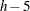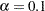Previous Page | Next Page

 The POWER Procedure

## Example 68.9 Binary Logistic Regression with Independent Predictors

Suppose you are planning an industrial experiment similar to the analysis in Getting Started: LOGISTIC Procedure of Chapter 51, The LOGISTIC Procedure, but for a different type of ingot. The primary test of interest is the likelihood ratio chi-square test of the effect of heating time on the readiness of the ingots for rolling. Ingots will be randomized independently into one of four different heating times (5, 10, 15, and 20 minutes) with allocation ratios 2:3:3:2 and three different soaking times (2, 4, and 6 minutes) with allocation ratios 2:2:1. The mass of each ingot will be measured as a covariate.

You want to know how many ingots you must sample to have a 90% chance of detecting an odds ratio as small as 1.2 for a five-minute heating time increase. The odds ratio is defined here as the odds of the ingot not being ready given a heating time ofminutes divided by the odds given a heating time ofminutes, for any time. You will use a significance level ofto balance Type I and Type II errors since you consider their importance to be roughly equal.

The distributions of heating time and soaking time are determined by the design, but you must conjecture the distribution of ingot mass. Suppose you expect its distribution to be approximately normal with mean 4 kg and standard deviation between 1 kg and 2 kg.

You are powering the study for an odds ratio of 1.2 for the heating time, but you must also conjecture odds ratios for soaking time and mass. You suspect that the odds ratio for a unit increase in soaking time is about 1.4, and the odds ratio for a unit increase in mass is between 1 and 1.3.

Finally, you must provide a guess for the average probability of an ingot not being ready for rolling, averaged across all possible design profiles. Existing data suggest that this probability lies between 0.15 and 0.25.

You decide to evaluate sample size at the two extremes of each parameter for which you conjectured a range. Use the following statements to perform the sample size determination:

```proc power;
logistic
vardist("Heat") = ordinal((5 10 15 20) : (0.2 0.3 0.3 0.2))
vardist("Soak") = ordinal((2 4 6) : (0.4 0.4 0.2))
vardist("Mass1") = normal(4, 1)
vardist("Mass2") = normal(4, 2)
testpredictor = "Heat"
covariates = "Soak" | "Mass1" "Mass2"
responseprob = 0.15 0.25
testoddsratio = 1.2
units= ("Heat" = 5)
covoddsratios = 1.4 | 1 1.3
alpha = 0.1
power = 0.9
ntotal = .;
run;
```

The VARDIST= option is used to define the distributions of the predictor variables. The distributions of heating and soaking times are defined by the experimental design, with ordinal probabilities derived from the allocation ratios. The two conjectured standard deviations for the ingot mass are represented in the Mass1 and Mass2 distributions. The TESTPREDICTOR= option identifies the predictor being tested, and the COVARIATES= option specifies the scenarios for the remaining predictors in the model (soaking time and mass). The RESPONSEPROB= option specifies the overall response probability, and the TESTODDSRATIO= and UNITS= options indicate the odds ratio and increment for heating time. The COVODDSRATIOS= option specifies the scenarios for the odds ratios of soaking time and mass. The default DEFAULTUNIT=1 option specifies a unit change for both of these odds ratios. The ALPHA= option sets the significance level, and the POWER= option defines the target power. Finally, the NTOTAL= option with a missing value (.) identifies the parameter to solve for.

Output 68.9.1 shows the results.

Output 68.9.1 Sample Sizes for Test of Heating Time in Logistic Regression
The POWER Procedure
Likelihood Ratio Chi-Square Test for One Predictor

Fixed Scenario Elements
Method Shieh-O'Brien approximation
Alpha 0.1
Test Predictor Heat
Odds Ratio for Test Predictor 1.2
Unit for Test Pred Odds Ratio 5
Nominal Power 0.9

Computed N Total
Index Response Prob Covariates Cov ORs Cov Units Total N Bins Actual Power N Total
1 0.15 Soak Mass1 1.4 1.0 1 1 120 0.900 1878
2 0.15 Soak Mass1 1.4 1.3 1 1 120 0.900 1872
3 0.15 Soak Mass2 1.4 1.0 1 1 120 0.900 1878
4 0.15 Soak Mass2 1.4 1.3 1 1 120 0.900 1857
5 0.25 Soak Mass1 1.4 1.0 1 1 120 0.900 1342
6 0.25 Soak Mass1 1.4 1.3 1 1 120 0.900 1348
7 0.25 Soak Mass2 1.4 1.0 1 1 120 0.900 1342
8 0.25 Soak Mass2 1.4 1.3 1 1 120 0.900 1369

The required sample size ranges from 1342 to 1878, depending on the unknown true values of the overall response probability, mass standard deviation, and soaking time odds ratio. The overall response probability clearly has the largest influence among these parameters, with a sample size increase of almost 40% going from 0.25 to 0.15.Previous Page | Next Page | Top of Page